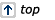# Electrical Formulas

More Formulas Conversion Formulas

Area of Circle = $$\pi r^2$$
Breakeven Dollars = Overhead Cost $/Gross Profit % Busbar Ampacity AL = 700A Sq. in. and CU = 1000A Sq. in. Centimeters = Inches x 2.54 Inch = 0.0254 Meters Inch = 2.54 Centimeters Inch = 25.4 Millimeters Kilometer = 0.6213 Miles Length of Coiled Wire = Diameter of Coil (average) x Number of Coils x $$\pi$$ Lightning Distance in Miles = Seconds between flash and thunder/4.68 Meter = 39.37 Inches Mile = 5280 ft, 1760 yards, 1609 meters, 1.609 km Millimeter = 0.03937 Inch Selling Price = Estimated Cost$/(1 - Gross Profit %)
Speed of Sound (Sea Level) = 1128 fps or 769 mph
Temp C = (Temp F - 32)/1.8
Temp F = (Temp C x 1.8) + 32
Yard = 0.9144 MetersElectrical Formulas Based on 60 Hz

Capacitive Reactance (Xc) in Ohms = 1/(2$$\pi$$ f C)
Effective (RMS) AC Amperes = Peak Amperes x 0.707
Effective (RMS) AC Volts = Peak Volts x 0.707
Efficiency (percent) = Output/Input x 100
Efficiency = Output/Input
Horsepower = Output Watts/746
Inductive Reactance (XL in Ohms = 2$$\pi$$ f L
Input = Output/Efficiency
Neutral Current (Wye) =$$\sqrt{A^2+B^2+C^2-(AB+BC+AC)}$$
Output = Input x Efficiency
Peak AC Volts = Effective (RMS) AC Volts x $$\sqrt 2$$
Peak Amperes = Effective (RMS) Amperes x $$\sqrt 2$$
Power Factor (PF) = Watts/VA
VA (apparent power) = Volts x Ampere or Watts/Power Factor
VA 1-Phase = Volts x Amperes
VA 3-Phase = Volts x Amperes x $$\sqrt 3$$
Watts (real power) Single-Phase = Volts x Amperes x Power Factor
Watts (real power) Three-Phase = Volts x Amperes x Power Factor x $$\sqrt 3$$Parallel Circuits

Note 1: Total resistance is always less than the smallest resistor
Note 1: RT = 1/(1/R1 + 1/R2 + 1/R3 +...)
Note 2: Total current is equal to the sum of the currents of all parallel resistors
Note 3: Total power is equal to the sum of power of all parallel resistors
Note 4: Voltage is the same across each of the parallel resistorsSeries Circuits

Note 1: Total resistance is equal to the sum of all the resistors
Note 2: Current in the circuit remains the same through all the resistors
Note 3: Voltage source is equal to the sum of voltage drops of all resistors
Note 4: Power of the circuit is equal to the sum of the power of all resistorsTransformer Amperes

Secondary Amperes 1-Phase = VA/Volts
Secondary Amperes 3-Phase = VA/(Volts x $$\sqrt 3$$)
Secondary Available Fault 1-Phase = VA/(Volts x %impedance)
Secondary Available Fault 3-Phase = VA/(Volts x $$\sqrt 3$$ x %Impedance)
Delta 4-Wire: Line Amperes = Phase (one winding) Amperes x $$\sqrt 3$$
Delta 4-Wire: Line Volts = Phase (one Winding) Volts
Delta 4-Wire: High-Leg Voltage (L-to-G) = Phase (one winding) Volts x 0.5 x $$\sqrt 3$$
Wye: Line Volts = Phase (one winding) Volts x $$\sqrt 3$$
Wye: Line Amperes = Phase (one winding) AmperesVoltage Drop

VD (1-Phase) = 2KID/CM
VD (3-Phase) = $$\sqrt 3$$ KID/CM
CM (1-Phase) = 2KID/VD
CM (3-Phase) = $$\sqrt 3$$ KID/VDCode Rules

Breaker/Fuse Ratings – 240.6(A)
Conductor Ampacity – 310.15 and Table 310.16
Equipment Grounding Conductor – 250.122
Grounding Electrode Conductor – 250.66
Motor Conductor Size – 430.22 (Single) 430.24 (Multiple)
Motor Short-Circuit Protection – 430.52
Transformer Overcurrent Protection – 450.3Constants

$$\pi$$(Pi) = (3.142 approximately)
$$\sqrt 2$$ = 1.414 (approximately)
$$\sqrt 3$$ = 1.732 (approximately)
f = Frequency